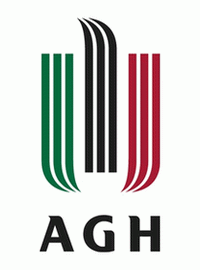Cracow, Poland# Faculty of Applied Mathematics

## Wydział Matematyki Stosowanej

Subject area: mathematics and statistics
University website: www.agh.edu.pl/en/
Mathematics has been present at the AGH University of Science and Technology since its origins. The first Rector of the University, professor Antoni Hoborski, was a mathematician. In 1969, the Departments of Mathematics and Descriptive Geometry were joined together to form the Institute of Mathematics, an interfaculty unit whose staff members taught at all university faculties. The institute became the Faculty of Applied Mathematics in 1997.
Applied Mathematics
Applied mathematics is the application of mathematical methods by different fields such as science, engineering, business, computer science, and industry. Thus, applied mathematics is a combination of mathematical science and specialized knowledge. The term "applied mathematics" also describes the professional specialty in which mathematicians work on practical problems by formulating and studying mathematical models. In the past, practical applications have motivated the development of mathematical theories, which then became the subject of study in pure mathematics where abstract concepts are studied for their own sake. The activity of applied mathematics is thus intimately connected with research in pure mathematics.
Faculty
Faculty may refer to:
Mathematics
Mathematics (from Greek μάθημα máthēma, "knowledge, study, learning") is the study of such topics as quantity, structure, space, and change. It has no generally accepted definition.
Applied Mathematics
From Pythagoras to Boethius, when pure mathematics consisted of arithmetic and geometry while applied mathematics consisted of music and astronomy, mathematics could be characterized as the deductive study of 'such abstractions as quantities and their consequences, namely figures and so forth' (Acquinas ca. 1260). But since the emergence of abstract algebra it has become increasingly difficult to formulate a definition to cover the whole of the rich, complex and expanding domain of mathematics.
George Frederick James Temple, 100 Years of Mathematics: a Personal Viewpoint (1981)
Applied Mathematics
My decision to leave applied mathematics for economics was in part tied to the widely-held popular belief in the 1960s that macroeconomics had made fundamental inroads into controlling business cycles and stopping dysfunctional unemployment and inflation.
Robert C. Merton, Robert C. Merton - Biographical at Nobelprize.org, 1995
Mathematics
A marveilous newtrality have these things mathematicall and also a strange participation between things supernaturall, imortall, intellectuall, simple and indivisible, and things naturall, mortall, sensible, compounded and divisible.
John Dee, The mathematicall praeface to the Elements of geometrie of Euclid of Megara (1570) as editor of Euclid's Elements, translated by Henry Billingsley.

## Contact:

30 Mickiewicza Av.
30-059 Krakow
Centre for International Students

Regular studies
P: +48 12 617 50 92
P: +48 12 617 46 15
F: +48 12 617 52 39
E: international.students@agh.edu.pl

Exchange programmes
P: +48 12 617 52 37
P: +48 12 617 52 38
F: +48 12 617 52 39
E: exchange@agh.edu.pl